## Holiday Discount at the Math Shop!

Seasons greetings math friends! Tis’ the season of giving, celebrating, and of course, glitter! And in honor of the holiday season, I’m here to take a break from providing math lessons this week, to instead, provide a holiday discount at the Math Shop! Did you know we have an exclusive math shop, just for us MathSux nerds? Check out the one-of-a-kind MathSux merchandise designs located in the Math Shop, found in the link below. Don’t forget to use the discount code, ‘CHEERSMATH‘ for a whopping 20% off! Also, check out my my top 3 favorite picks below for a quick preview of what you’ll find in the store. Does anyone else have nerdy math gear that they use in their lives and in their classroom? Let me know in the comments below!

## Holiday Discount:

Math Shop: https://mathsux.creator-spring.com/

Discount: ‘CHEERSMATH

The Math Shop has one-of-a-kind math themed designs on t-shirts, stickers, and posters in a variety of colors for all the math nerds in your life. These items are perfect for math fans, teachers, and students to keep studying the subject light and positive! This can be perfect for those frustrated learning moments, for example, quotes like “Keep Calm and Calculate on” will carry any math learner through to the more enlightened side of math. Although this was hard to do, check out my personal top 3 favorites from the Math Shop:

1) Peace, Love, Pi T-Shirt in Pink:

2) MathSux Stickers

3) Keep Calm and Calculate on Stickers

Hoping you’re all having a wonderful holiday season and a Happy New Year! Last but not least, of course I also want to wish you happy calculating! Be back with more math lessons in 2022!

## NYS Regents Review – Algebra June 2021

Greetings math friends! Today we are going to break down the NYS Regents, specifically the Algebra NYS Common Core Regents from June 2021, one question at a time. The following video playlist goes over each and every question one step at a time. I’ve been working on this playlist slowly adding new questions and videos every week and now that it is complete, it is time to celebrate (and/or study)! Please enjoy this review along with the study aids and related links that will also help you ace the NYS Regents. Happy calculating!

## Study Resources:

Looking to ace your upcoming NYS Regents!? Don’t forget to check out the resources below, including an Algebra Cheat Sheet, and important topics and videos to review. Good luck and happy calculating!😅

Algebra Cheat Sheet

Combing Like Terms and the Distributive Property

How to Graph an Equation of a Line

Piecewise Functions

NYS Regents – Algebra 2020

## How to Study Math?

How is one supposed to study math!? Well, there is usually only one way, and that is to practice, practice, practice! But don’t get too stressed, because you can also make practice fun (or at least more pleasant).

Add some background music to your study session and make a nice cup of tea before diving in for the brain marathon. Another idea is to study only 1 hour at a time and to be sure to take breaks. Can’t seem to get that one question? Take a break and walk a way, or even better find a new study spot! It’s been scientifically proven that studying in different places can boost your memory of the very information you’re trying to understand.

What study habits do you have that have worked for you in the past? Let me know in the comments and good luck on your upcoming test!

** Be sure to check out the full list of Algebra lessons and old Regents questions review!**

## How to Construct an Equilateral Triangle?: Geometry

Happy Wednesday math peeps! This post introduces constructions by showing us how to construct an equilateral triangle by using a compass and straightedge (or ruler). For anyone new to constructions in geometry, this is the perfect topic for art aficionados since there is more drawing here than there is actual math!

## What is an Equilateral Triangle?

Equilateral Triangle: An equilateral triangle is a triangle with three equal sides.  Not an easy one to forget, the equilateral triangle is super easy to construct given the right tools (compass+ straightedge). Take a look below:

## Construct an Equilateral Triangle Example:

Check out the GIF below to see how to construct an equilateral triangle step by step using a compass and straightedge with pictures and explanations below!

## What’s Happening in this GIF?

1. Using a compass, we measure the distance of line segment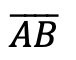.

2. With the compass point remaining on point A, we then draw an arc that has the same distance as line segment.

3. With the compass now placed on point B, draw an arc that has the same distance as line segment.

4. Notice where the arcs intersect? Using a ruler, connect points A and B to the new intersection point. This will create two new equal sides of our triangle!

5. We have now officially constructed a triangle with all equal side lengths!

## Constructions and Related Posts:

Looking to construct more than just an equilateral triangle? Check out these related posts on geometry constructions!

Construct a Perpendicular Bisector

Perpendicular Line through a Point

Angle Bisector

Construct a 45º angle

Altitudes of a Triangle (Acute, Obtuse, Right)

Construct a Square inscribed in a Circle

## Best Geometry Tools!

Looking to get the best construction tools? Any compass and straight-edge will do the trick, but personally, I prefer to use my favorite mini math toolbox from Staedler. Stadler has a geometry math set that comes with a mini ruler, compass, protractor, and eraser in a nice travel-sized pack that is perfect for students on the go and for keeping everything organized….did I mention it’s only \$7.99 on Amazon?! This is the same set I use for every construction video in this post. Check out the link below and let me know what you think!

Still got questions? No problem! Don’t hesitate to comment with any questions. Happy calculating! 🙂

Looking to learn more about triangles? Check out this post on right triangle trigonometry here! And if you’re looking for even more geometry constructions, check out the link here!

## Expanding Cubed Binomials: Algebra 2/Trig.

Greetings math friends! This post will go over expanding cubed binomials using two different methods to get the same answer. We’re so used to seeing squared binomials such as,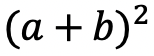, and expanding them without a second thought.  But what happens when our reliable squared binomials are now raised to the third power, such as,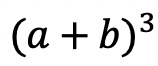?  Luckily for us, there is a Rule we can use:

But where did this rule come from?  And how can we so blindly trust it? In this post we will prove why the above rule works for expanding cubed binomials using 2 different methods:

Why bother? Proving this rule will allow us to expand and simplify any cubic binomial given to us in the future! And since we are proving it 2 different ways, you can choose the method that best works for you.

## Method #1: The Box Method

Step 1: First, focus on the left side of the equation by expanding (a+b)3:

Step 2: Now we are going to create our first box, multiplying (a+b)(a+b). Notice we put each term of (a+b) on either side of the box. Then multiplied each term where they meet.

Step 3: Combine like terms ab and ab, then add each term together to get a2+2ab+b2.

Step 4: Multiply (a2+2ab+b2)(a+b) making a bigger box to include each term.

Step 5: Now combine like terms (2a2b and a2b) and (2ab2 and ab2), then add each term together and get our answer: a3+3a2b+3ab2+b3.

## Method #2: The Distribution Method

Let’s expand the cubed binomial using the distribution method step by step below:

Now that we’ve gone over 2 different methods of cubic binomial expansion, try the following practice questions on your own using your favorite method!

Practice Questions: Expand and simplify the following.

Solutions:

Still, got questions?  No problem! Check out the video above or comment below! Happy calculating! 🙂

**Bonus: Test your skills with this Regents question on!

## Recursive Rule

Welcome to Mathsux! This post is going to show you everything you need to know about how to use a Recursive Formula by looking at three different examples of a recursive rule. Check out the video below for more of an explanation and test your skills with the practice questions at the bottom of this page.  Please let me know if you have any questions in the comments section below and happy calculating! 🙂

## What is a Recursive Formula or a Recursive Rule?

A Recursive Formula is a type of formula that forms a sequence based on the previous term value.  The recursive rules for each formula vary, but we are always given the first term and a formula to find the consecutive terms in the recursive sequence.

Recursive formula can be written as an arithmetic sequence (a sequence where the same number is either added or subtracted to each subsequent term to form a pattern) and recursive formulas can also be written as arithmetic sequences (a sequence where the same number is either multiplied or divided to each subsequent term to form a pattern). We’ll go over an example of each but both types of recursive rules are treated the same exact way!

What does all of this mean?  Check out the example below for a clearer picture.

## Example #1: Arithmetic Recursive Sequence

Step 1: First, let’s decode what these formulas are saying.

Step 2: The first term, represented by a1, is and will always be given to us. In this case, our first term has the value a1=2 and represents the first term of our recursive sequence.

a1= First Term=2

Step 3: We then plug in the value of our first term, which is a1=2 into our formula an+4 to get 2+4=6. The number 6 now has the value of our second term in the recursive sequence.

a1= 2 First Term

a2= (2)+4=6 Second Term

Step 4: Now we are going to continue the pattern, plugging in the value of each previous term to find the next consecutive terms in our recursive sequence.

The pattern can be more easily seen below. Notice we are able to find the value of all 5 terms of the recursive sequence for the solution only given the first term and recursive formula at the beginning of our question.

Step 5: We found the recursive sequence we were looking for: 2, 6, 10, 14, 18. Since the question was originally only asking for the value of the fifth term we know our solution only needs to be the value of the fifth term which is 18.

## Example #2: Geometric Recursive Sequence

Step 1: First, let’s decode what these formulas are saying.

Step 2: The first term, represented by a1, is and will always be given to us. In this case, our first term has the value a1=1 and represents the first term of our recursive sequence.

a1= First Term=1

Step 3: We then plug in the value of our first term, which is a1=1 into our formula 2an+1 to get 21+1=3. The number 3 now has the value of our second term in the recursive sequence.

a1= 1 First Term

a2= 2(1)+1=3 Second Term

Step 4: Now we are going to continue the pattern, plugging in the value of each previous term to find the next term in our recursive sequence.

The pattern can be more easily seen below. Notice we are able to find the value of all 3 terms of the recursive sequence for the solution only given the first term and recursive formula at the beginning of our question.

***Note this was written in a different notation but is solved in the exact same way! This recursive formula is a geometric sequence.

Step 5: We found the recursive sequence we were looking for: 1,3,9. Since the question was originally only asking for the value of the third term we know our solution only needs to be the value of the third term which is 9.

## Example #3:

Step 1: First, let’s decode what these formulas are saying.

Step 2: The first term, represented by a1, is and will always be given to us. In this case, our first term has the value a1=4 and represents the first term of our recursive sequence.

a1= First Term=4

Step 3: We then plug in the value of our first term, which is a1=4 into our formula 3an-1-2 to get 3(2)-1=5. The number 5 now has the value of our second term in the recursive sequence.

a1= 4 First Term

a2= 3(2)-1=5 Second Term

Step 4: Now we are going to continue the pattern, plugging in the value of each previous term to find the next term in our recursive sequence.

The pattern can be more easily seen below. Notice we are able to find the value of all 3 terms of the recursive sequence for the solution only given the first term and recursive formula at the beginning of our question.

Step 5: We found the recursive sequence we were looking for: 4,10,28. Since the question was originally only asking for the value of the third term we know our solution only needs to be the value of the third term which is 28.

Think you are ready to solve a recursive equation on your own?! Try finding the specific term in each given recursive function below:

## Related Posts:

Looking to learn more about sequences? You’ve come to the right place! Check out these sequence resources and posts below. Personally, I recommend looking at the arithmetic sequence or geometric sequence posts next!

Arithmetic Sequence

Geometric Sequence

Finite Arithmetic Series

Finite Geometric Series

Infinite Geometric Series

Golden Ratio in the Real World

Fibonacci Sequence

Still, got questions? No problem! Don’t hesitate to comment below or reach out via email. And if you would like to see more MathSux content, please help support us by following ad subscribing to one of our platforms. Thanks so much for stopping by and happy calculating!

***Bonus! Want to test yourself with a similar NYS Regents question on Recursive Formulas?  Click here! And if you want to learn about even more sequences, check out the link here!

## Reflections: Geometry

Greetings and welcome to Mathsux! Today we are going to go over reflections geometry, one of the many types of transformations that come up in geometry. And thankfully, reflection transformation is one of the easiest types to master, especially if you’re more of a visual learner/artistic type person. In this post, we will go over a reflection across the line x=-2, but if you want to see a reflection across the x axis, the y axis, and the line y=x, please check out the math video below! So let’s get to it! And if you’re new to transformations, check out translations here.

## What are Reflections?

A Reflection on a coordinate plane is exactly what you think! A reflection is a type of transformation in geometry where we reflect a point, a line segment, or a shape over a line to create a mirror image of itself.  Think of the wings of a butterfly, a page being folded in half, or anywhere else where there is perfect symmetry, each of these are great examples of a reflection!

## Reflection Rules:

If you are completely new to reflections, you may want to check out the video above that goes into deriving reflection rules over the different axis. But for those of you more comfortable with reflection, check out the example below where we reflect the image of a triangle onto itself over the line x=-2.

## Example:

Step 1: First, let’s draw in line x=-2. Note that whenever we have x equal to a number, we end up drawing a vertical line at that point on the x axis, in this case at x=-2.

Step 2: Find the distance each point is from the line x=-2 and reflect it on the other side, measuring the same distance.

First, let’s look at point C, notice it’s 1 unit away from the line x=-2 on the right. To reflect point c, we are going to count 1 unit but this time to the left of the line x=-2 and label our new point, C|.

Step 3: Now we are going to reflect coordinate point A in much the same way! Notice that point A is 2 units away on the left of line x=-2, we then want to measure 2 units to the right of our line x=-2 and mark our new coordinate point, A|.

Step 4: Lastly, we want to reflect coordinate point B. This time, point B is 1 unit away on the right side of the line x=-2, we then measure 1 unit to the opposite direction of our line, x=-2, and mark our new point, B|.

Step 5: Now that we have all the newly reflected coordinate points of our triangle, finally, we can now connect them all, for our fully reflected image of right triangle A|B|C|.

Notice our newly reflected triangle is not just a mirror image of itself, but when the original figure is reflected it actually ends up overlapping onto itself!? How did this happen? That is because this our reflection line came right down the middle of our original image, triangle ABC. Shapes that reflect onto themselves are a bit tricky but not impossible, just remember to measure out the distance of each coordinate point and reflections should be a breeze!

## Rigid Motion:

Reflections are a special type of transformation in geometry that maintains rigid motion, meaning when a point, line, or shape is reflected the angles, and line segments retain their value. For example, if we were to measure the area of both right triangles, before and after reflection, we would find the areas to remain unchanged. Meaning the area of triangle ABC is equal to the area of triangle A|B|C| . Another rigid transformation includes rotations and translations.

Looking to practice your new reflection skills? Try the reflection practice problems below, with solutions to each question, to truly master the topic! Happy calculating!

## Solutions:

Still got questions?  No problem! Check out the video above or comment below! There is also a bonus video if you scroll all the way down at the end of this post for anyone who wants to see how to reflect a line over the line x=2. See how it differs and how it is similar to the example shown here. Happy calculating! 🙂

Don’t forget to follow MathSux on social media for the latest tips and tricks to ace math!

And if you are looking for more Transformations Check out the related posts below and let me know if you have any questions? Or maybe you just want to share your favorite type of transformation? Either way, happy calculating!

Translations

Dilations

Rotation

## Piecewise Functions: Algebra

Greetings, today’s post is for those in need of a piecewise functions review!  This will cover how to graph each part of that oh so intimidating piecewise functions.  There’s x’s, there are commas, there are inequalities, oh my! We’ll figure out what’s going on here and graph each part of the piecewise-function one step at a time.  Then check yourself with the practice questions at the end of this post. Happy calculating! 🙂

## What are Piece-Wise Functions?

Exactly what they sound like! A function that has multiple pieces or parts of a function.  Notice our function below has different pieces/parts to it.  There are different lines within, each with their own domain.

Now let’s look again at how to solve our example, solving step by step:

Translation: We are going to graph the line f(x)=x+1 for the domain where x > 0

To make sure all our x-values are greater than or equal to zero, we create a table plugging in x-values greater than or equal to zero into the first part of our function, x+1.  Then plot the coordinate points x and y on our graph.

Translation: We are going to graph the line  f(x)=x-3 for the domain where x < 0.

To make sure all our x-values are less than zero, let’s create a table plugging in negative x-values values leading up to zero into the second part of our function, x-3.  Then plot the coordinate points x and y on our graph.

## Practice Questions:

Graph each piecewise function:

## Solutions:

Still got questions?  No problem! Check out the video above or comment below for any questions. Happy calculating! 🙂

***Bonus! Want to test yourself with a similar NYS Regents question on piecewise functions?  Click here.

## Intersecting Secants Theorem: Geometry

Ahoy! Today we’re going to cover the Intersecting Secants Theorem!  If you forgot what a secant is in the first place, don’t worry because all it is a line that goes through a circle.  Not so scary right? I was never scared of lines that go through circles before, no reason to start now.

If you have any questions about anything here, don’t hesitate to comment below and check out my video for more of an explanation. Stay positive math peeps and happy calculating! 🙂

Wait, what are Secants?

Intersecting Secants Theorem: When secants intersect an amazing thing happens! Their line segments are in proportion, meaning we can use something called the Intersecting Secants Theorem to find missing line segments.  Check it out below:

Let’s now see how we can apply the intersecting Secants Theorem to find missing length.

Step 1: First, let’s write our formula for Intersecting Secants.

Step 2: Now fill in our formulas with the given values and simplify.

Step 3: All we have to do now is solve for x! I use the product.sum method here, but choose the factoring method that best works for you!

Step 4: Since we have to reject one of our answers, that leaves us with our one and only solution x=2.

Practice Questions: Find the value of the missing line segments x.

Solutions:

Still got questions?  No problem! Check out the video above or comment below for any questions and follow for the latest MathSux posts. Happy calculating! 🙂

To review a similar NYS Regents question check out this post here.

## Circle Theorems & Formulas

Inscribed Angles & Intercepted Arcs

Area of a Sector

Circle Theorems

## Absolute Value Equations: Algebra

Happy Wednesday math friends! Today, we’re going to go over how to solve absolute value equations.  Solving for absolute value equations supplies us with the magic of two potential answers since absolute value is measured by the distance from zero.  And if this sounds confusing, fear not, because everything is explained below!

Also, if you have any questions about anything here, don’t hesitate to comment. Happy calculating! 🙂

Absolute Value measures the “absolute value” or absolute distance from zero.  For example, the absolute value of 4 is 4 and the absolute value of -4 is also 4.  Take a look at the number line below for a clearer picture: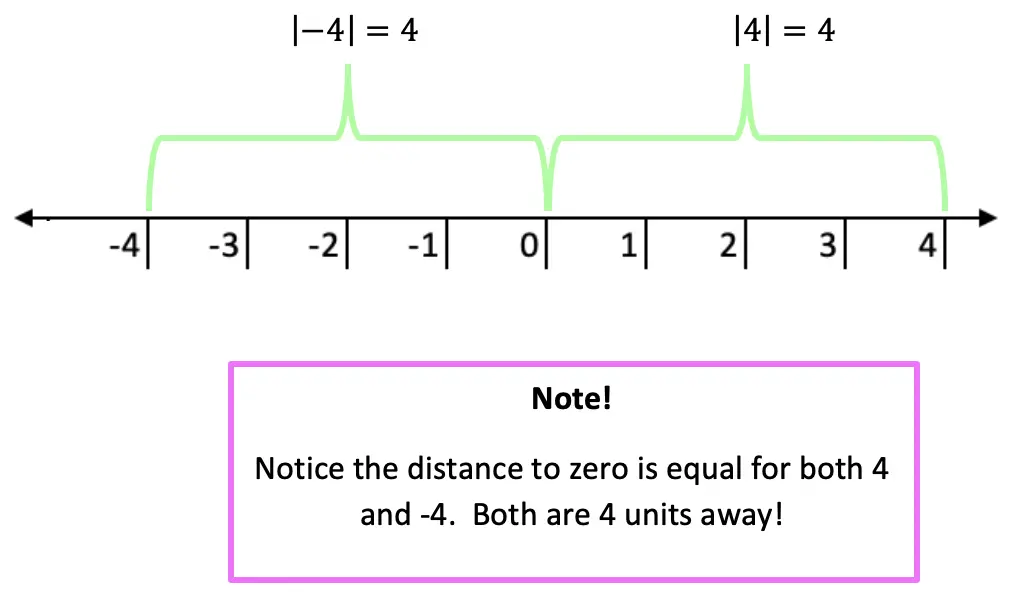Now let’s see how we can apply our knowledge of absolute value equations when there is a missing variable!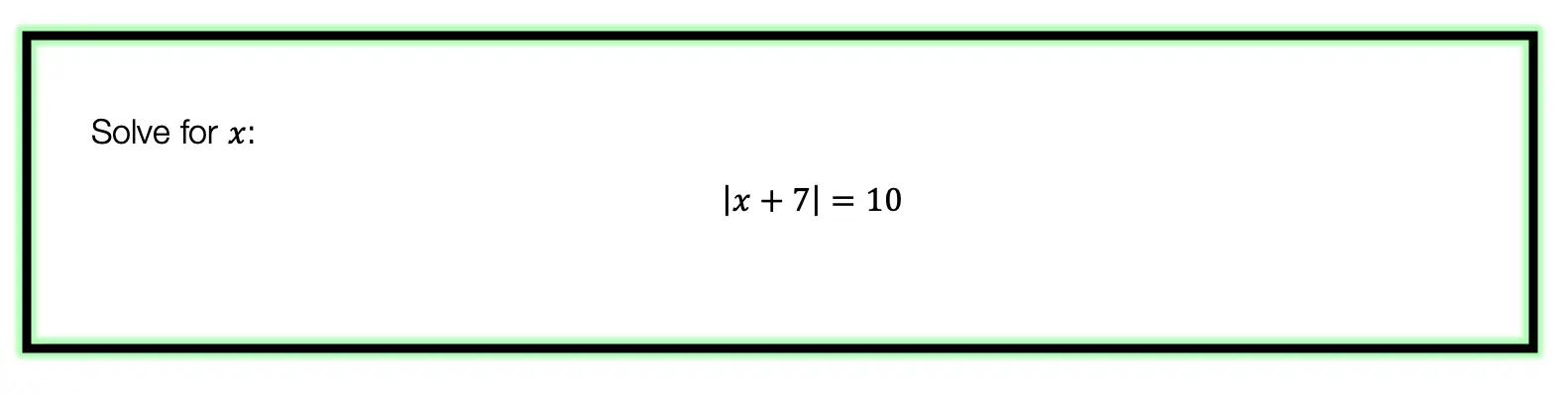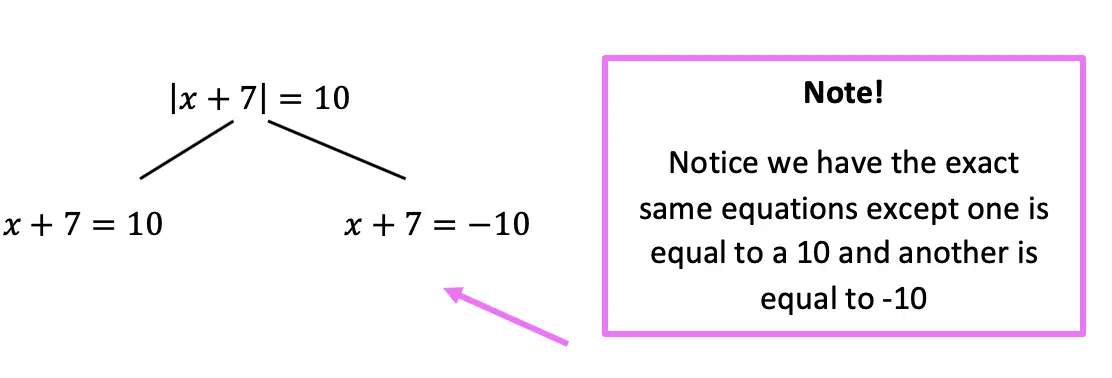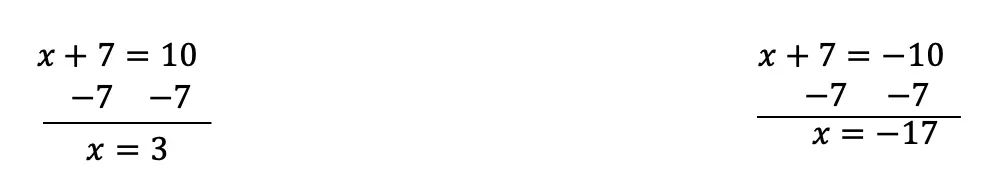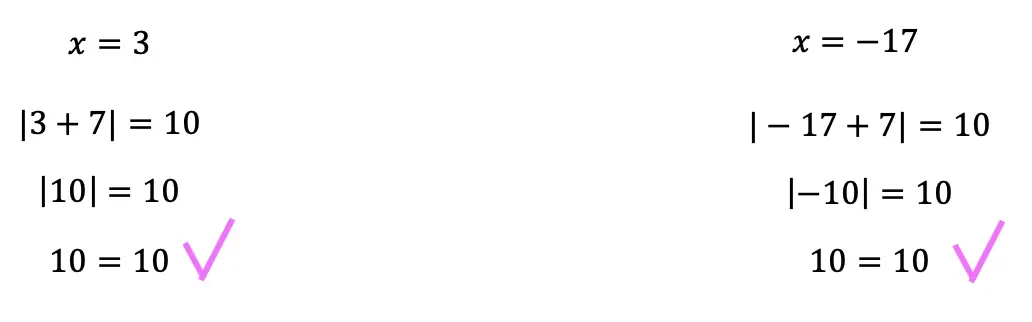Now let’s look at a slightly different example: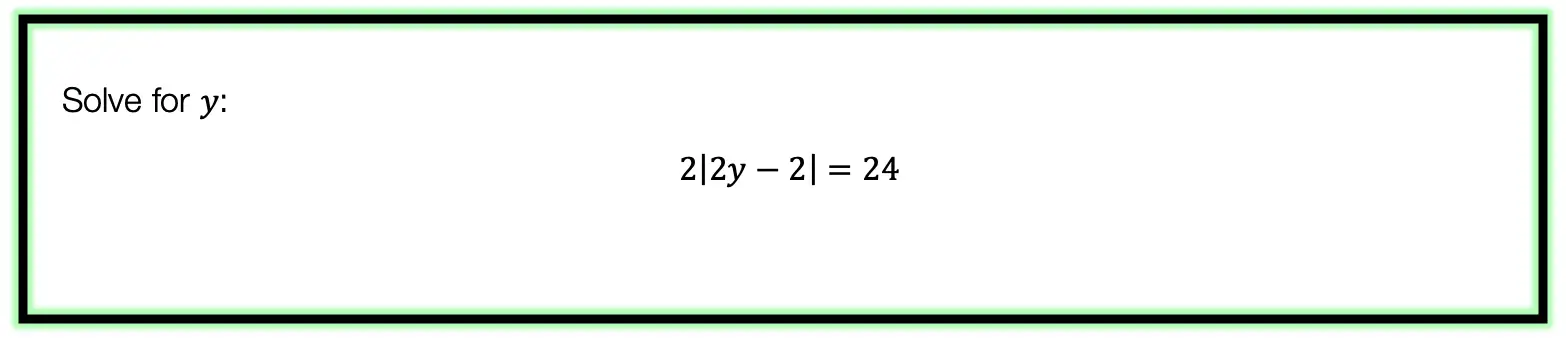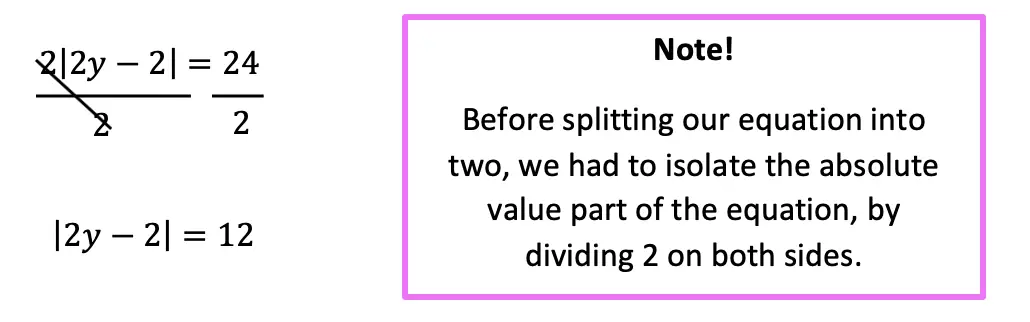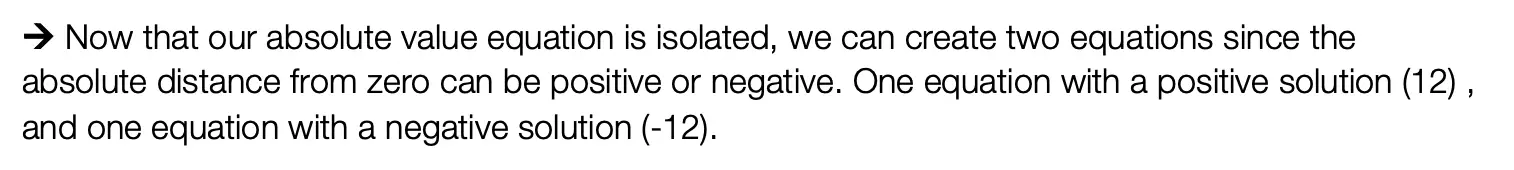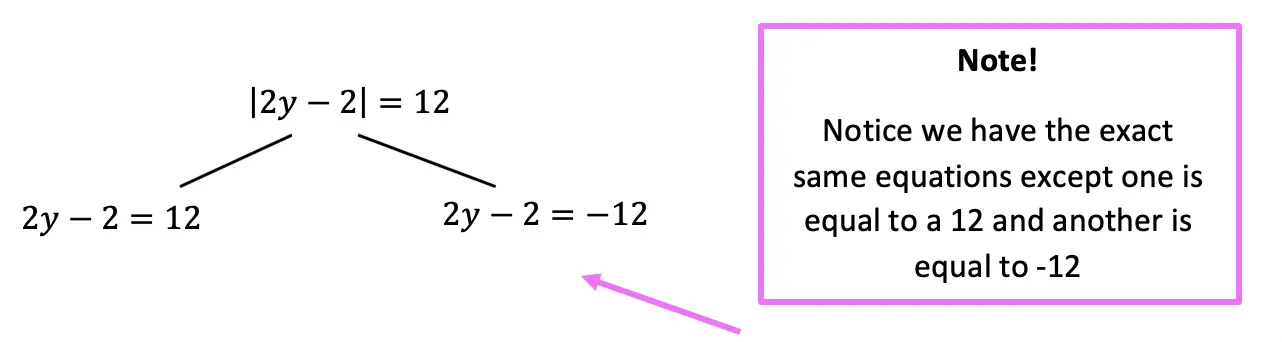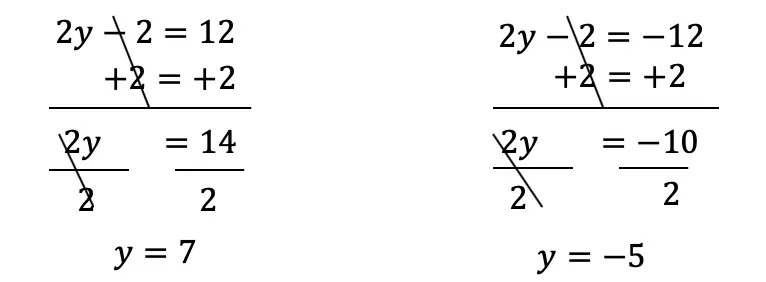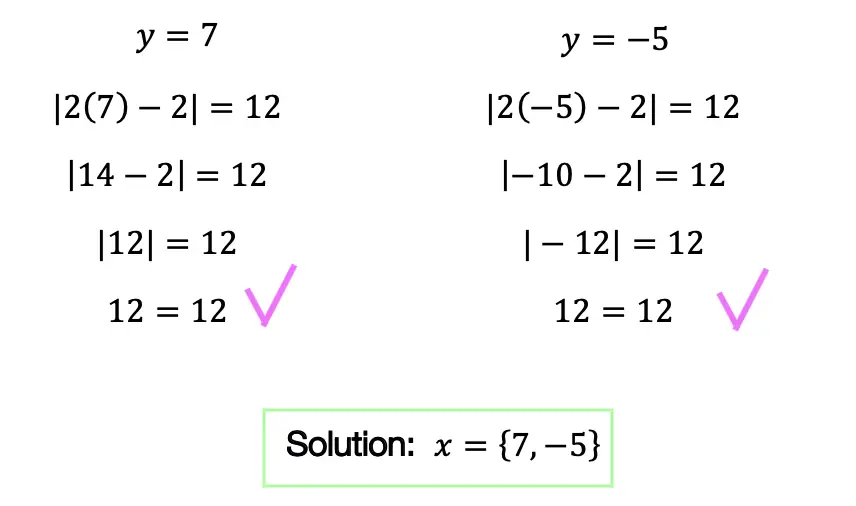Practice Questions: Given the following right triangles, find the missing lengths and side angles rounding to the nearest whole number.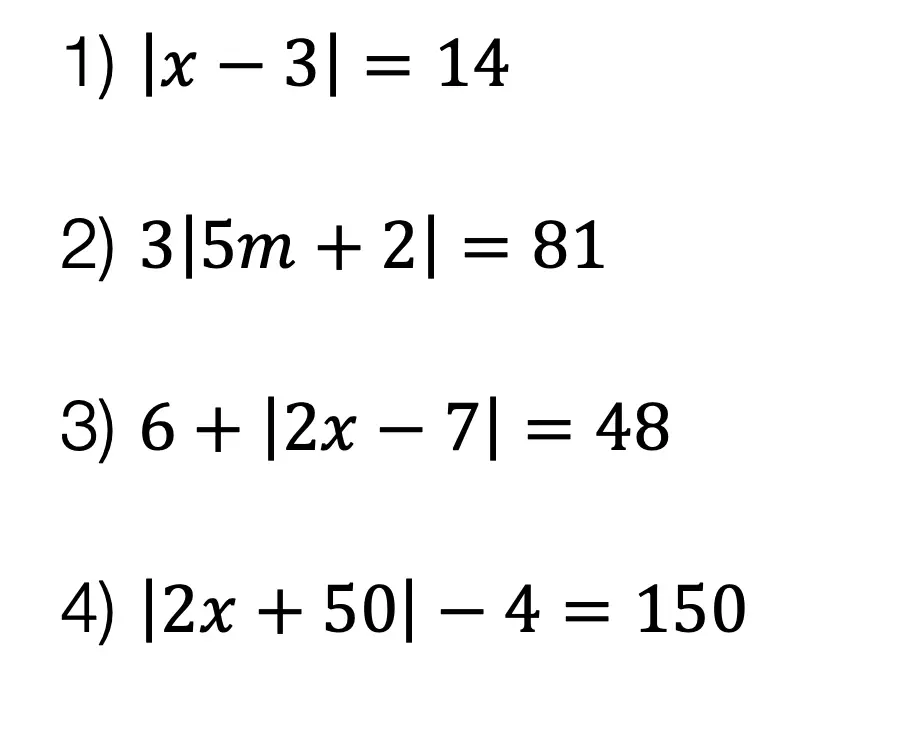Solutions: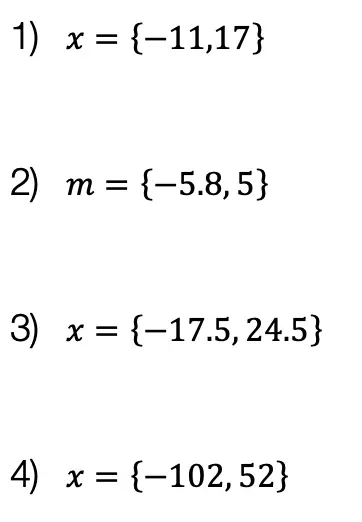Still got questions?  No problem! Check out the video the same examples outlined above. Happy calculating! 🙂

Also, if you’re looking for a review on combining like terms and the distributive property, check out this post here.

## How to use SOH CAH TOA: Geometry

Greeting math friends and welcome to Mathsux! In this post, we are going to start with the very basics of trigonometry by going over how to find a missing angle and/or side length of right triangles while using the famous trigonometric function sine, cosine, or tangent, (aka how to use SOH CAH TOA).  Woo hoo! These are the basics of right triangle trigonometry, and provide the base for mastering so many more interesting things to come in trigonometry! So, let’s get to it!

SOH CAH TOA is an acronym that stands for the following trig functions and parts of a right triangle. We’ll explain more in this post!

SOH CAH TOA

Also, if you have any questions about anything here, don’t hesitate to comment below or watch the video below. Also, don’t forget to subscribe to MathSux for FREE math videos, lessons, and practice questions every week. Happy calculating! 🙂

## What does SOH CAH TOA stand for?

A Trigonometric Ratio, more commonly known as Sine, Cosine, and Tangent, are trig ratios that naturally exist within a right triangle.  This means that the sides and angles of a right triangle are in proportion within itself.  It also means that if we are missing a side or an angle of a right triangle, based on what we’re given, we can figure out what the value of the sides or angles are, based on these ratios!

Let’s take a look at what Sine, Cosine, and Tangent are all about!

Ready for your first right angled triangle example? Check it out below!

## SOH CAH TOA Example #1:

Now let’s see how we can apply trig ratios when there is a missing side or angle in a right triangle!

Step 1: First, let’s identify the different sides of our right triangle depending on which angle we are focusing on, which in this case is a 60º angle. Based on the locations of our angle, we can label each side as the hypotenuse, adjacent, or the opposite.

Notice below, that the opposite side labeled x, is labeled the “opposite” side because it is opposite to our given angle, 60º. The remaining side is considered the adjacent side of our triangle because it is directly next to our given angle, 60º.

Step 2: Now, let’s write out SOH CAH TOA. Notice the only trig function that uses both the hypotenuse and the opposite is sine! Knowing to use the sine function, let’s fill in our formula using the hypotenuse = 5 and opposite = x in order to find the value for missing side length x.

In order to use the sin function correctly, we’re going to need to plug in our given angle, which is 60º, and then set up our proportion. sin(60º)=x/5. By using the sine function, our calculator, and a little bit of algebra we’ll be able to solve for the unknown side.

Ready for another example?! Check out another SOH CAH TOA problem using right triangles below!

## Right Triangle Trig Example #2:

Step 1: First, let’s identify the different parts of the right triangle we are given (the hypotenuse, adjacent, and the opposite). Notice in this example, we are given the adjacent and hypotenuse and need to find the value of the unknown angle, θ.

Step 2: Next, let’s write out our acronym, SOH CAH TOA, to see which trig function can help us with our question! Notice the only trig function that uses both adjacent and hypotenuse is cosine. This is what we will use to solve for the unknown angle, θ.

We use cosine, by setting up our proportion, cos(θ)=adjacent/ hypotenuse, knowing we can then plug in 12 for our adjacent value, and 13 for our hypotenuse value.

Think you’re ready to test out SOH CAH TOA on your own? Try the following Practice Questions on your own!

## Practice Questions:

Given the following right triangles, find the missing lengths and side angles rounding to the nearest whole number.

## Solutions:

Still got questions?  No problem! Check out the video the same examples outlined above and happy calculating! 🙂

## Related Trigonometry Posts:

The Unit Circle

Basic Right Triangle Trigonometric Ratios (SOH CAH TOA)

4545 90 Special Triangles

30 60 90 Special Triangles

Graphing Trig Functions

Transforming Trig Functions

Factoring Trig Functions

Law of Cosines

Trig Identities

Law of Sines